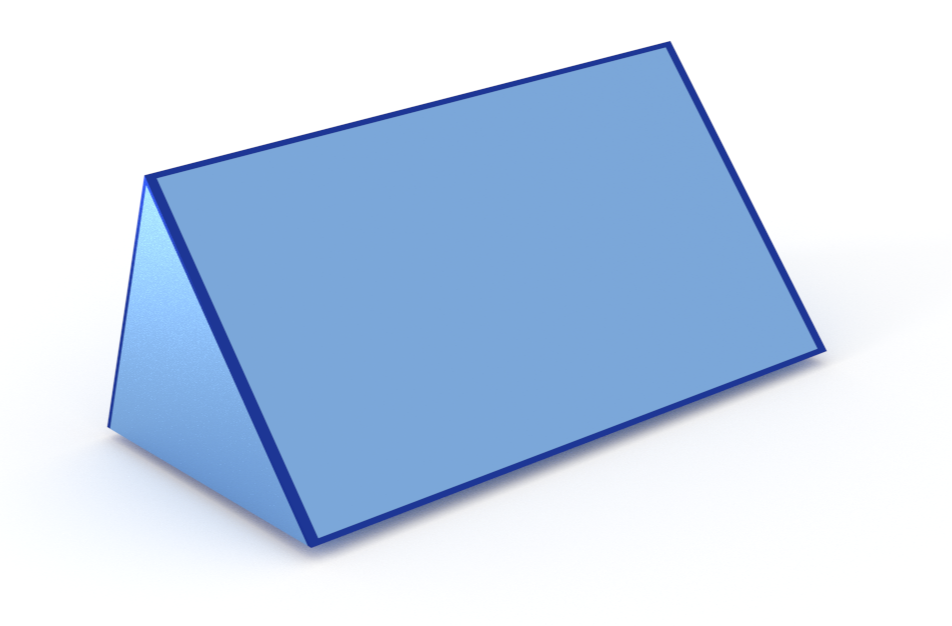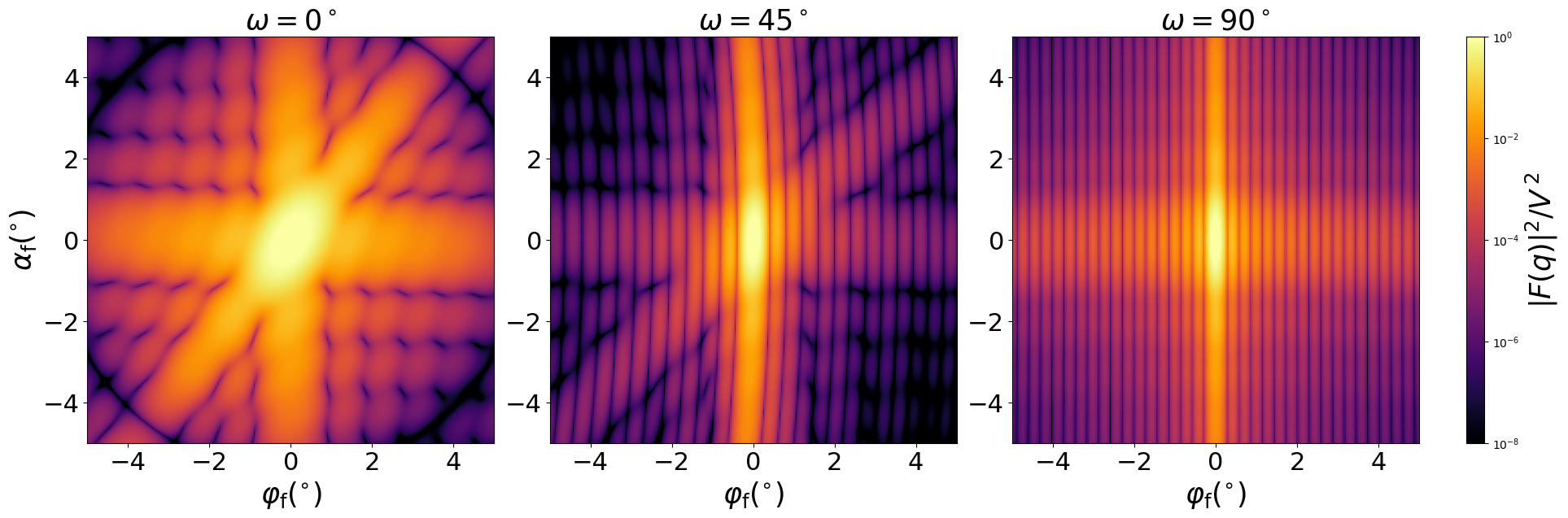## Ripples

Elongated particles, or ripples, are typically used to model lamellar cuts or gratings.

As everywhere else in BornAgain only single scattering in the DWBA is simulated. This can be insufficient for periodic gratings that cause noticeable higher-order diffraction. But for the foreseeable future, multiple-scattering effects are not in the scope of BornAgain.

We choose ripples to be elongated in $x$ direction. Different profiles in the $YZ$ plane can be set, for example, bar, cosine, sawtooth. For each of them, different profiles can also be chosen in the $XZ$ plane.

The approach to setting the ripple profiles will be changed soon

### Example

A ripple with an asymmetric saw-tooth profile that is uniform in $x$-direction.#### Constructor

SawtoothRippleBox(L, W, H, d)


Parameters:

• L, length
• W, width
• H, height
• d, asymmetry

Constraint:

$|d| \le W/2$

#### Usage

As for any other Form factor.

#### Implementation

Class SawtoothRipple inherits from the interface class IFormFactor .

Form factor is computed as

$$F(\mathbf{q}) = f_\parallel(q_x) i\text{e}^{-i q_y d} \left[ \text{e}^{i \alpha_{-}/2} \text{sinc}\left( \frac{\alpha_{+}}{2} \right) - \text{e}^{i \alpha_{+}/2} \text{sinc}\left( \frac{\alpha_{-}}{2} \right) \right],$$

with the notation $$\alpha_{+} = H q_z + \frac{q_y W}{2} + q_y d, \quad \alpha_{-} = H q_z - \frac{q_y W}{2} + q_y d.$$

The longitudinal factor $f_\parallel$ is defined according to “box shape” in $XZ$ plane:

$$f_\parallel(q_x)=L \space \text{sinc}(q_xL/2).$$

Volume has been validated against $$V=\dfrac{LWH}{2}.$$

#### Other shapes

We have already stated that transversal $YZ$ and longitudinal $XZ$ profiles are set separately. For other longitudinal shapes use

SawtoothRippleGauss(L, W, H, d)
SawtoothRippleLorentz(L, W, H, d)


with the corresponding factor $f_\parallel(q_x)$ inside.

Other transversal profiles are also available:

cosine profile

CosineRippleBox(L, W, H)
CosineRippleGauss(L, W, H)
CosineRippleLorentz(L, W, H)


and rectangular profile

Box(L, W, H)
BarGauss(L, W, H)
BarLorentz(L, W, H)


#### Scattering

Scattering by uncorrelated, oriented sawtooth ripples for horizontal incidence. Rotation around $z$ axis:Generated by Examples/ff/SawtoothRippleBox.py .

#### History

“CosineRippleBox” and “SawtoothRippleBox” replicate “Ripple1” and “Ripple2” from FitGISAXS [Babonneau 2013].

Full documentation and API support for all ripple form factors appeared in BornAgain 1.17. Before that release, the Lorentz factor $f_\parallel$ had an extra factor of 2.5. The Gauss factor $f_\parallel$ was completely wrong up through BornAgain 20.